Math Apps
 App Description App Description App DescriptionSketch2graph Category - Education Learn more about the relationship between the graphical and mathematical representation of math expressions. You draw the graph and get back the math that expresses what you drew. Rating - ___ out of 5 stars Cost - FreeGeometry Pad+ Category - Education Creat efundmaental geometric shapes, change their properties and calculate metrics. Shapes displayed on a scrollable and zoomable workbook with rectangular coordinate system. Include text annotations. Use the transformation tool and manualklky input coordinates. Rating - ___ out of 5 stars Cost - \$5.99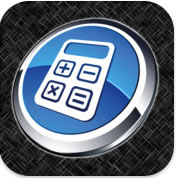Calculator XL Category - Utilities Takes advantage of the ipad's large screen. Has all the common calculator functions including memory on exit. Rating - 4.5 out of 5 stars Cost - Free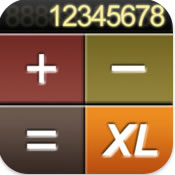Calculator XL Category - Productivity A standard (some extra functions), scientific (30 different functions), unit (length, weight, temperature - Imperial and US), tip (3 tip rates) and Loan calculator (month and year amortization). Rating - 4.5 out of 5 stars Cost - \$1.99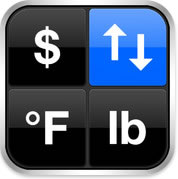Convert Any Unit Free Category - Utilities 5,300 units in 41 categories.Search for and select units with autocomplete, automatic category selection, flip units button and reset button to clear all inputs. Rating - 4.5 out of 5 stars Cost - Free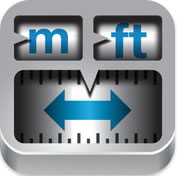Converter - Unit and Currency Category - Productivity Built in calculator, convert over 400 units across 16 categories, convert multiple units, save favorite conversions, invert the conversion, currency rates auto-update and simple search tools. Rating - 5 out of 5 stars Cost - \$3.99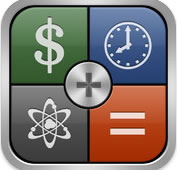Converter Plus Category - Utilities A unit converter with an extensive list of currencies and categories such as area, weight, energy, force, length, mass, power, etc. Simultaneous multiple conversions and support for compound units. Rating - 4.5 out of 5 stars Cost - \$1.99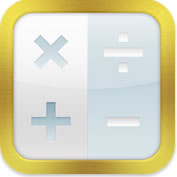Solver for iPad Category - Productivity Write out calculations line by line (words along side numbers) on a notepad on the left and answers displayed on the right. Multiline calculations and work is automatically saved. Rating - 4 out of 5 stars Cost - \$4.99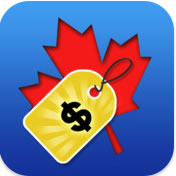My Price (Canada) Category - Finance Easily and quickly calculate Canadian sales tax and discounts for every province. Rating - 4.5 out of 5 stars Cost - Free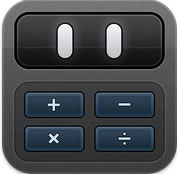Calcbot - The Intellegent Calculator Category - Utilities Simple user interface and accessible advanced functions with live as-you-type view. Calculation history tape. Rating - 4.5 out of 5 stars Cost - \$1.99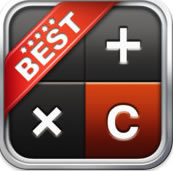Calculator Category - Utilities Provides all scientific and arithmetic functions and covers operations such as factorial, exponential, root, logarithm and trig functions. Expression view allows you to keep track of calculations. Rating - 4.5 out of 5 stars Cost - \$1.99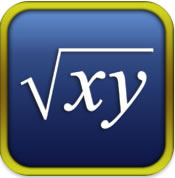Symbolic Calculator HD Category - Education Exact arithmetic, extensive algebra (solve equations, systems) and calculus)integrals, derivatives) capabilities, formula display in natural form, store results, unlimited number of variables and user defined functions. Rating - 4 out of 5 stars Cost - \$1.99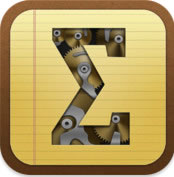Scalar Category - Productivity An endless sheet of paper so you never lose track of a calculation. Automatic save. Editable entries with automatic recalculation. Entries can be referenced from each other. Rating - 4.5 out of 5 stars Cost - \$4.99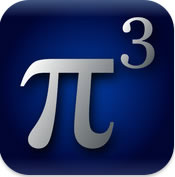Pi Cubed Category - Education Let syou construct, typeset and instantly evalaute mathematical expressions. Supports log, trig functions, roots, exponents and factorials. Has a built-in database of 150 equations. Rating - 4 out of 5 stars Cost - \$9.99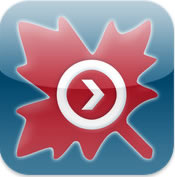Maple Player Category - Education Comes with a collection of interactive calculators and conceptual explorations. Find solutions to integrals, derivatives, limits, plot arbitrary functions, andcalculate solutions to linear systems. Rating - 4.5 out of 5 stars Cost - FreeMathStudio Category - Education Full featured calculator with over 200 functions. Covers topics in algebra, calculus (limits, derivatives, integrals), statistics (scatter, histograms, box), and graphing (2D, 3D Time). Rating - 4.5 out of 5 stars Cost - \$19.99Quick Graph Category - Education Displays explicit and implicit equations and inequalities in both 2D and 3D in a coordinate, cartesian, polar, spherical or cylindrical plane. Display up to 6 equations at one time and evaluate equaions at specific points. Rating - 4.5 out of 5 stars Cost - Free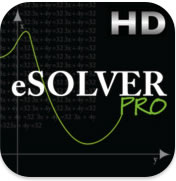eSolver HD Category - Education Solve quadratic equations by entering the coefficients. Get instant soultion for f(x) = 0, and evaluate f(x) and f'(x) for a different "x". Rating - 3 out of 5 stars Cost - FreeWolfram Algebra Category - Education Evaluate numeric expression or sbstitutea value for a variable, simplify frations radicals, solve simple equations and systems, plot basic and parametric functions, expand or factor polynomials, divide two expressions and partial fraction decomposition. Rating - 4 out of 5 stars Cost - \$1.99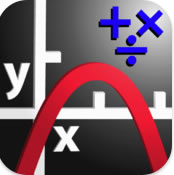Free Graphing Calculator Category - Education A scientific calculator, a graphing calculator (4 equations at one time, graphs labelled, find roots and intersections), a unit convertor, constant for scientific calculations, table of values and a reference library. Rating - 5 out of 5 stars Cost - Free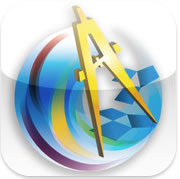Sketchpad Explorer Category - Education Drag, manipulate and animate visual mathematics to develop and generalize fundamental concepts. Rating - 5 out of 5 stars Cost - Free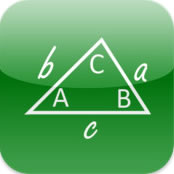Triangle Solver Category - Education Solve triangles if you know 2 angles and one side, 2 sides and one angle or 3 sides and no angles. Rating - 3.5 out of 5 stars Cost - FreeiMathematics! Category - Education 700 formulas, definitions and theorems with a search function and 100 quizzes. Has a scientific, graphic and advanced calculator; fraction approximator; systems and matrices solver, and a math glossary. Rating - 4.5 out of 5 stars Cost - FreeNoteCalc Category - Education Displays formula and the result at the same time as if written on a notepad. Allows altering and correction of data at any time. Four variables to store constants, save unlimited number of calculations, 20 levels of parentheses and accuracy to 15 digits. Rating - 4.5 out of 5 stars Cost - \$1.99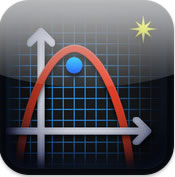Quadratic Master Category - Education Quadratic equation and function solver and formulas. Detailed solution, information and factorization. Solve 4 types of inequality. Solve quadratic functions in three forms - general, vertex and factored. 3D animation of rotating cone. Rating - 3.5 out of 5 stars Cost - \$1.99Statistics toolkit Category - Education Computes all basic statistics including mean, weighted mean, sample and population standard deviation, correlation, combinations, permutations, probability and others. Perform forecasting Rating - 3out of 5 stars Cost - \$1.99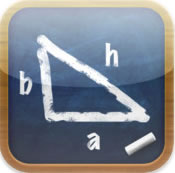iFormulas Category - Education Categories include: algebra, calculus, geometry and trig. Save tab bar rearrangments, favorite formuals, and scrollable and zoomable views Rating - 3 out of 5 stars Cost - Free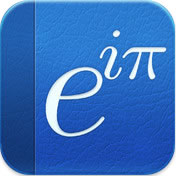Math Ref Category - Education Browse over 1,400 formulas, figures and examples. Helpful tools such as unit converter, quadratic solver and triangle solver. Features: save, search, zoom and editable notes. Rating - 3.5 out of 5 stars Cost - \$1.99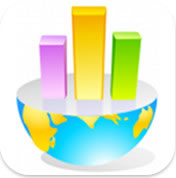Statistics Visualizer Category - Education A comprehensive statistical data analysis tool providing dynamic graphs and calculations with detailed explanantions. free version provides Ninomial, Poisson, Geometric, Normal, Chi-Square, exponential distributions. Rating - 4 out of 5 stars Cost - Free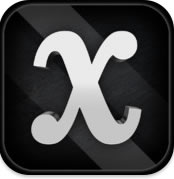Algerba Touch Category - Education Refresh your skills. Switch between lessons and randomly-generated practice problems. Use an equation editor to create your own set of problems. Covers topics such as: simplification, orders of operation, factorization, basic equations, factoring. Rating - 4.5 out of 5 stars Cost - \$1.99PreCalculus Category - Education A library of videos allowing students to leverage a wide range of topics. The videos work through a numerous set of problems. Rating - 3.5 out of 5 stars Cost - FreeGeometry Category - Education A library of videos allowing students to leverage a wide range of topics. The videos work through a numerous set of problems. Rating - 4 out of 5 stars Cost - FreePreAlgebra Category - Education A library of videos allowing students to leverage a wide range of topics. The videos work through a numerous set of problems. Rating - ___ out of 5 stars Cost - Free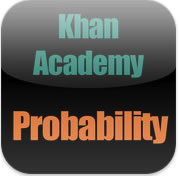Probability Category - Education A library of videos allowing students to leverage a wide range of topics. The videos work through a numerous set of problems. Rating - 4 out of 5 stars Cost - Free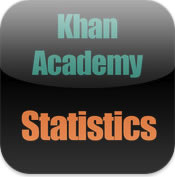Statistics Category - Education A library of videos allowing students to leverage a wide range of topics. The videos work through a numerous set of problems. Rating - 4 out of 5 stars Cost - Free